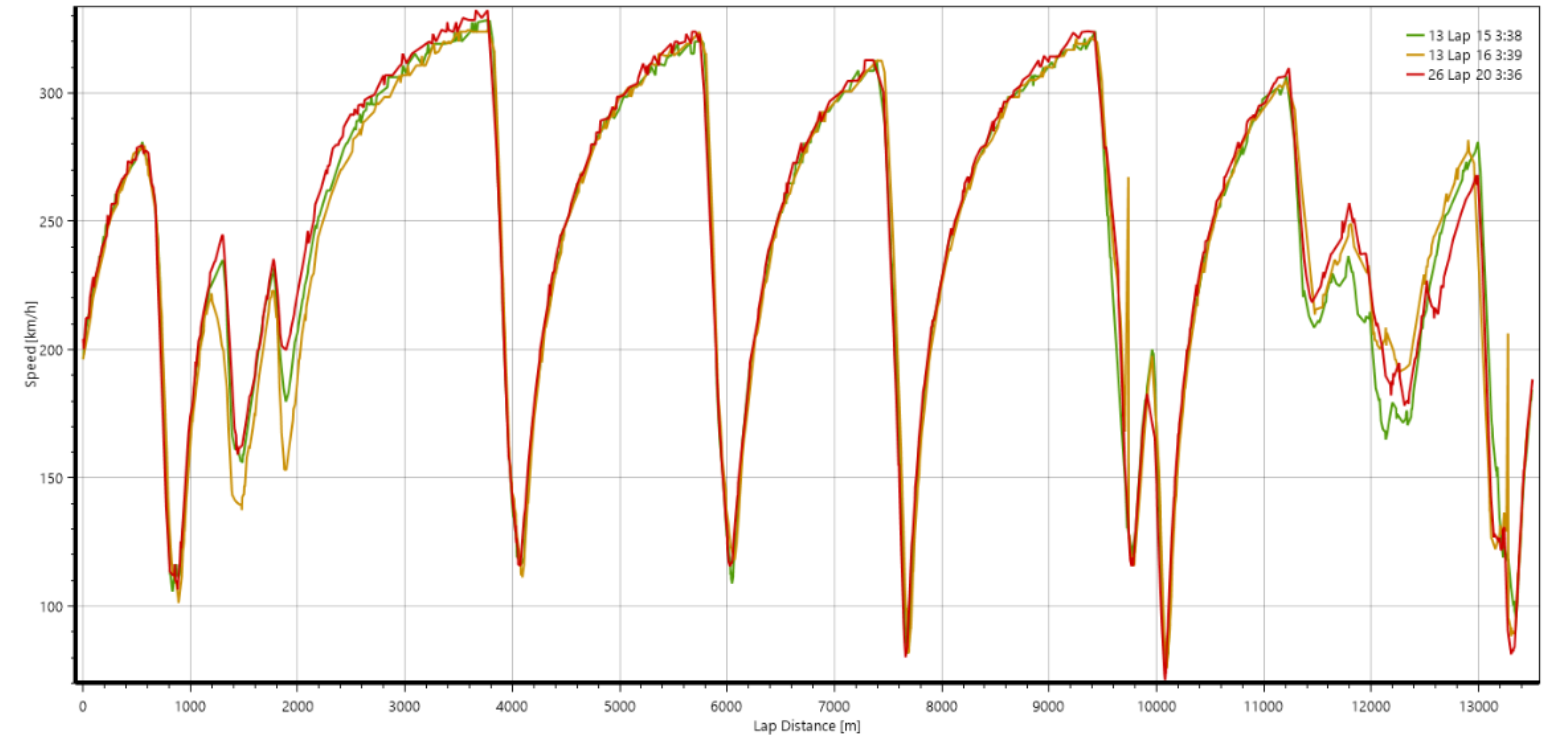# Plotting

## Scatter

A large number of parameters are available for plotting on a scatter graph with both X and Y axes fully definable. The parameters available for both axes are shown below.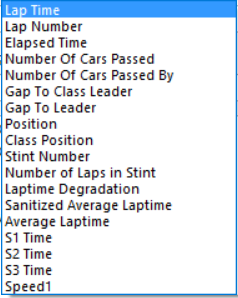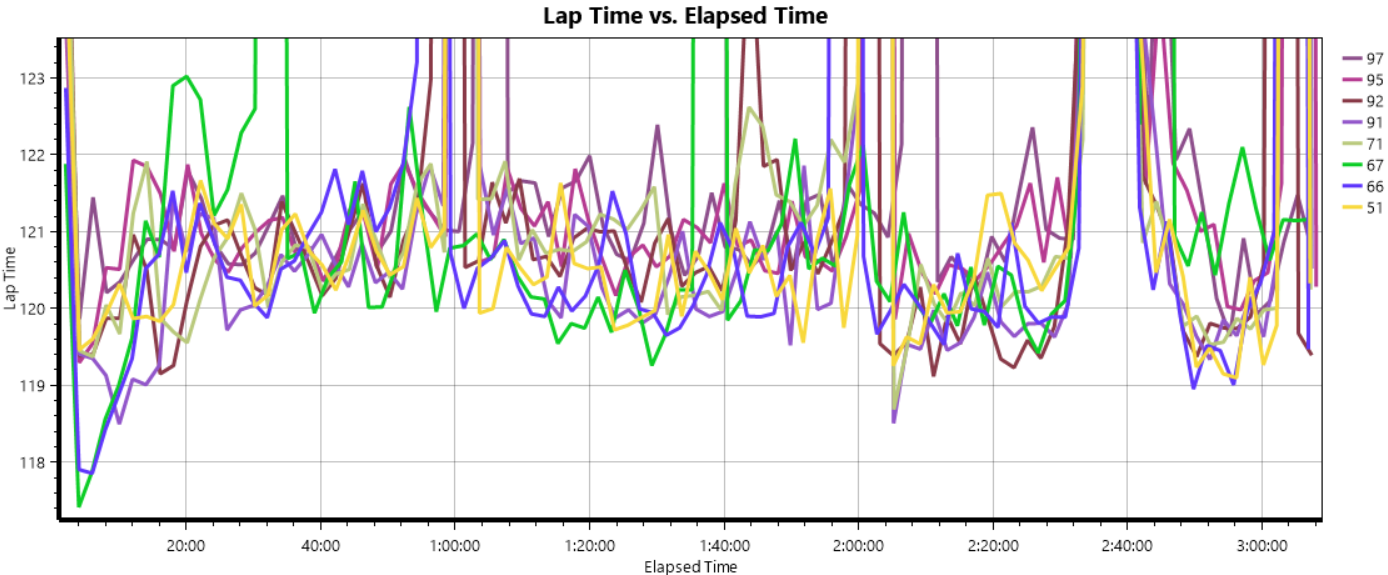Filers can be added to the plot series to only look at ranges of interest. For example of you wanted to remove the In and Out laps from a lap time plot an upper limit can be applied via the Edit Series button in the ribbon control as shown below.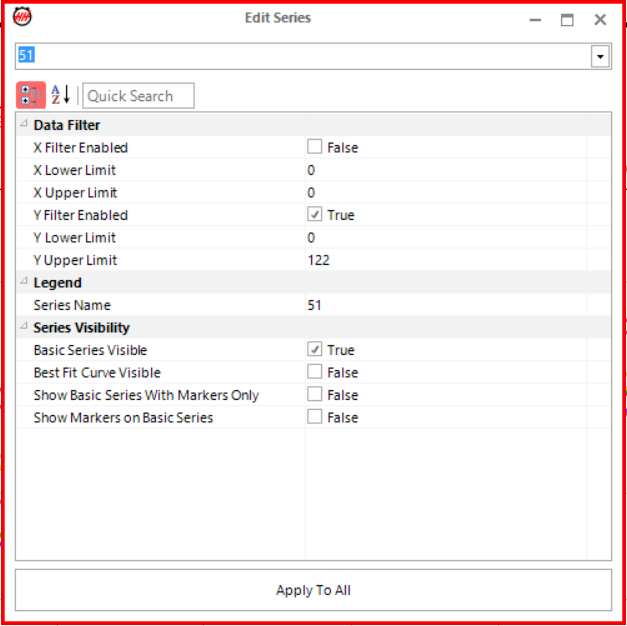## Raising Average

The raising average graph can be used to analyse practice/qualifying sessions or a race. The graph is a plot of the average best X laps for different values of X, starting from 1. In the example plot, for the lap number 60 the corresponding y-value shows the average lap time of the best 60 laps for each car. The raising average graph also gives an indication of the consistency of each car/driver. The shallower the slope the more consistent a car/driver is over the corresponding number of laps shown on the x-axis. The raising average plot is useful to see the overall performance of a car/driver in a session, but does not allow for analysis of individual stints or contiguous subsets of laps.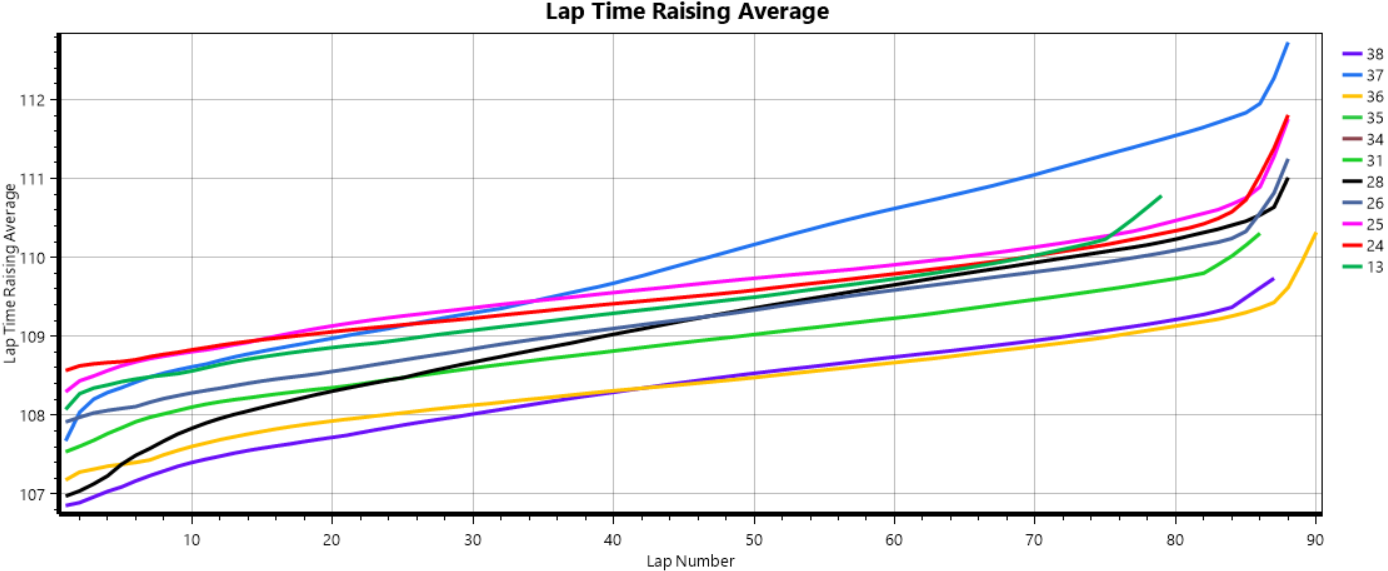## Gap Graph

The gap graph is only used to analyse a race and shows the gap between a reference and other cars in the field. On the y-axis the gap to the reference is shown in seconds, and on the x-axis the lap number of the race is shown. Positive y-values indicate that a car is behind the reference, and negative y-values indicate that a car is ahead of the reference.

The gap chart is useful for analysing the pace of the reference car over periods of laps (e.g. stints and/or the whole race) relative to the other cars shown on plot. Because a number of laps are studied there is a filtering effect that gives a much clearer picture of the relative pace of the two cars compared with looking at raw lap time data.

### Reference Modes

There are 3 difference modes that can be used to set the reference as shown below.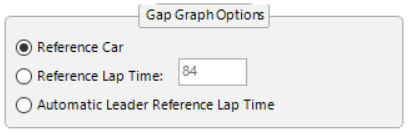#### Reference Car

The gap of every car relative to a chosen reference car at the end of each lap.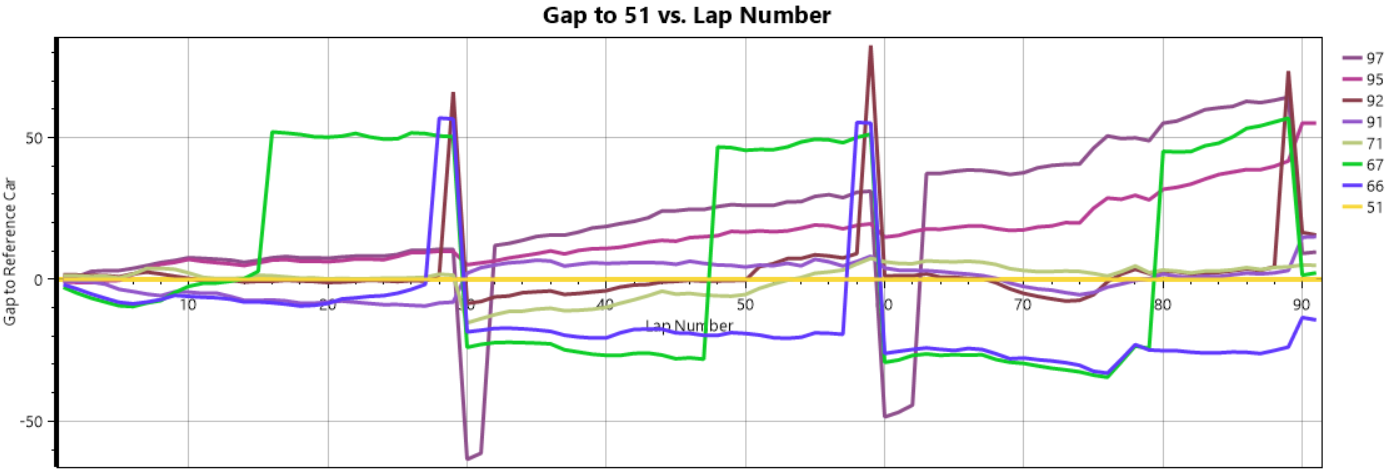#### Reference Lap Time

The gap of every car relative a lap time. This can be thought of as the gap to a hypothetical car that does the exact same lap time for the entire race.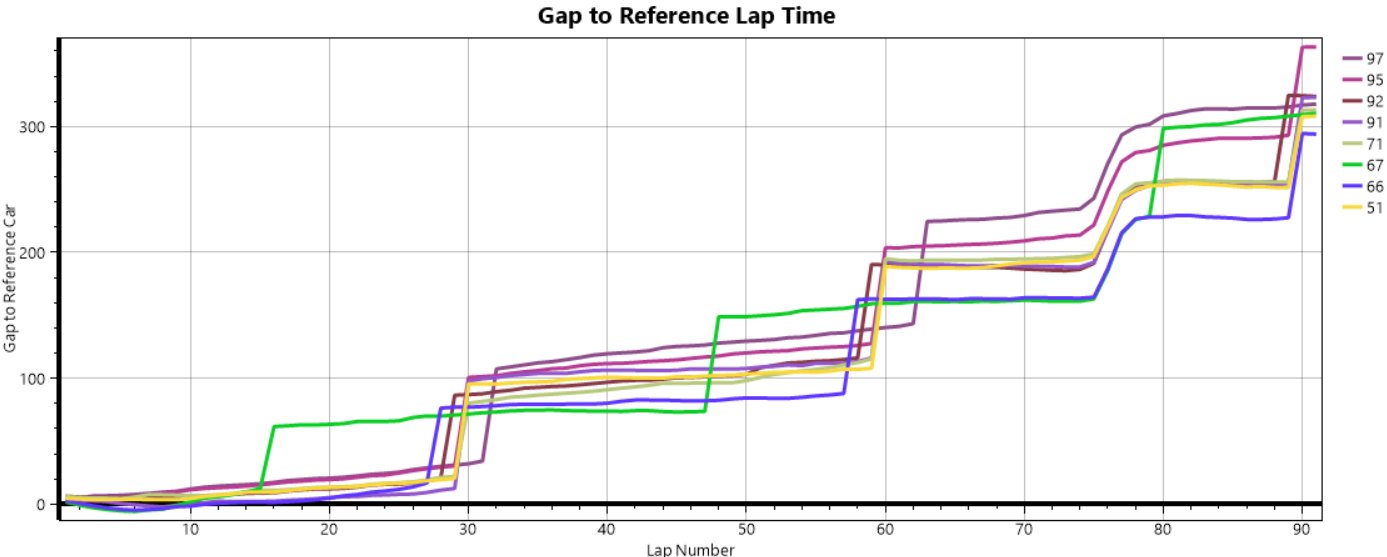#### Automatic Leader Reference Lap Time

A class is selected, the lap time of the current leader for that class is then set as the reference for the whole race.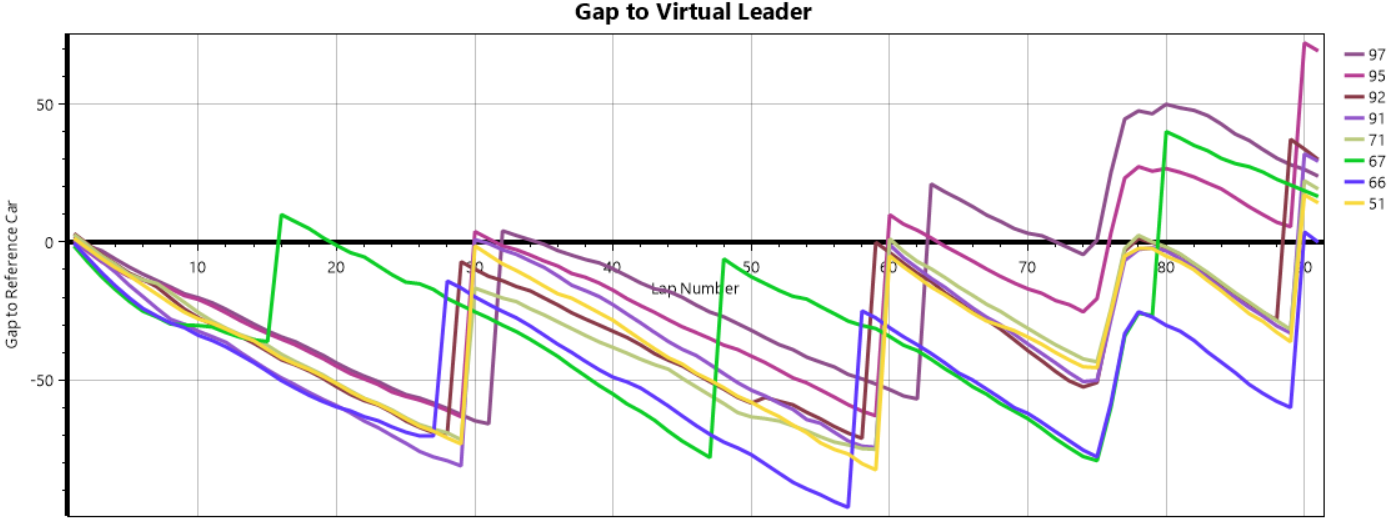## Projected Gap Graph

Similar to the normal gap graph for a reference car with the addition of prediction until the end of the race. The actual gap to the car in question is shown up to the current point in the race which is denoted with the vertical red line. The assumed future pace for each car is the average of the best 3 laps in the last 5 laps. The pit stop sequence for the forward prediction can be based on the  Estimated Stint Lengths or Fixed Stint Lengths depending on what is appropriate.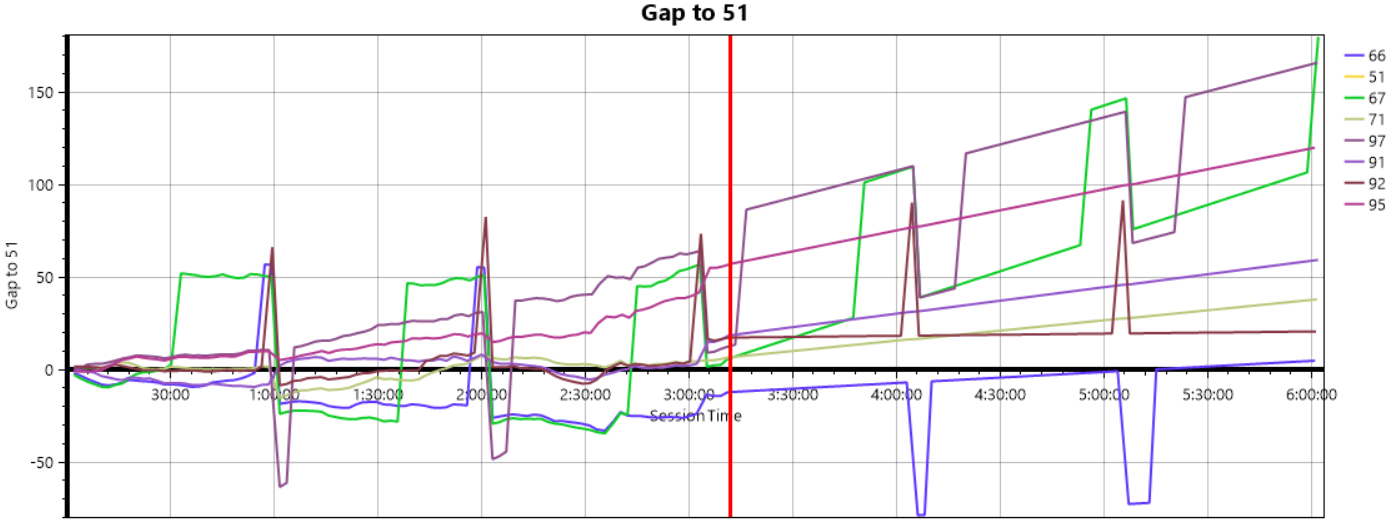## GPS Speed

In series where GPS data is available over the timing feed (such as WEC and Formula E) this data can be visualized in HH Timing. The speed traces and position on track (driving lines) of each car can be shown.

Note that this data is based on taking the derivative of position data which has two main sources of error:

1. Error in the GPS position measurement
2. Numerical error from taking the derivative

These errors can be large enough that the data is of little use for determining speed. In order to determine the speed via GPS to a reasonable accuracy the speed signal from the GPS unit on the car must be used which is not given in the data feed.